## Indicators

•Cited by SciELO
•Access statistics

•Cited by Google
•Similars in SciELO
•Similars in Google

## Print version ISSN 0012-7353On-line version ISSN 2346-2183

### Dyna rev.fac.nac.minas vol.78 no.166 Medellín Apr./June 2011

RADIATION MODEL FOR PREDICTING TEMPERATURE EVOLUTION IN SOLAR COOKER

MODELO DE RADIACIÓN PARA PREDECIR LA EVOLUCIÓN DE LA TEMPERATURA EN COCINAS SOLARES

FARID CHEJNE
Universidad Nacional de Colombia, Medellín, fchejne@unal.edu.co

ANDRÉS MACÍA
Universidad Nacional de Colombia, Medellín, afmacia@gmail.com

Universidad Nacional de Colombia, Medellín, afmacia@gmail.com

HECTOR IVAN VELÁSQUEZ
Universidad Nacional de Colombia, Medellín, hivelasq@unal.edu.co

CARLOS LONDOÑO
Universidad Nacional de Colombia, Medellín, clondono@unal.edu.co

Received for review December 10th, 2009, accepted October 8th, 2010, final version, November 4th, 2010

ABSTRACT: A mathematical model that describes and simulates the thermal behavior of a solar stove was developed based on an electric resistances analogy. The mathematical model includes the three different heat transfer mechanisms between different surfaces of the solar stove and its environment. The mathematical model was used to predict the solar stove entropy generation and its efficiency; also, it was used to evaluate the design parameters of a solar box stove. Experimental and theoretical data were compared and found to be satisfactory.

KEYWORDS: Solar energy, cooker, mathematical model, entropy.

RESUMEN: Un modelo matemático que describe y simula el comportamiento térmico de una estufa solar fue desarrollado en base en la analogía de resistencias aléctricas. El modelo matemático incluye los tres diferentes mecanismos de transferencia de calor entre las diferentes superficies de la cocina solar y su entorno. El modelo matemático se utilizó para predecir la generación de entropía y de su eficiencia; tambien, fue utilizado para evaluar los parametros de diseño de una estufa solar tipo caja. Los datos experimentales y teóricos fueron comparados satisfactoriamente.

PALABRAS CLAVE: Cocina solar, radiación, analogía eléctrica.

1. INTRODUCTION

The solar stove is a perfect example of appropriate technology that provides alternatives to improve the quality of life of hundreds of people in Colombia [1,2]. The non-uniform distribution of energy resources in many Colombian regions , together with the lack of appropriate public utilities (light, water, sewer system, and telephone), necessitates the study of this type of alternative technology [4,5].Passamai and co-workers made an updated bibliographical survey of the development of solar box-type stoves. Additionally, they developed a mathematical model that predicts the thermal behaviour of such devices ; Mohamad and Kader analyzed the effects of several designs of solar stove [7-9]. Johnson and Edwards developed a method based on finite difference to simulate a solar stove with sensitive storage materials [10,11]; Winston developed a constructive principle of ideal concentrators based on the marginal rays method [12,13].

2. DESCRIPTION OF THE SOLAR COOKER

Figure 1 shows a schematic of a box-type solar cooker with a cooking container made in the Universidad Nacional de Colombia (Medellín).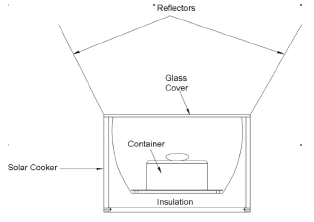Figure 1.
Schematic illustration of a typical box solar stove

The cooker consists of a stainless steel tray (30 cm · 40 cm at bottom, 49 cm · 49 cm at top, and 26 cm height) made of a 0.3 mm thick sheet. The conventional container (B 20 cm) was loaded with a fixed mass of thermal fluid (1L). The sides and bottom of the tray are encased in a box made of wood. The gap between the tray and the casing is filled with polyurethane to provide thermal insulation. The tray is provided with a movable glass cover, hinged to one side of the casing at the top. A plane glass mirror, encased in a sheet metal shell, is fitted to serve as a reflector. This serves as a cover for the glass glazing when the cooker is not in use. The cooking container is conventional and has a flat base. The container is provided with tight fitting covers. The outer sides of the vessels are painted with dull black paint.

3. MATHEMATIC MODELLING

The development of a mathematical model is presented for a solar box-type stove. The model is based on the case of a box with a container inside.

1.1 Box-Stove
The mathematical model developed here is a radiation heat transfer model for a box-type stove with a container inside (See Fig. 2), which would receive solar energy. It is assumed that all the surfaces are grey and fuzzy, that absorptivity and emissivity are constant with temperature, the emitted and reflected radiation are distributed fuzzily, the flux of the outgoing energy is distributed uniformly on the entire surface, the floor is considered an adiabatic surface, the insulation is considered as a volumetric configuration with uniform distribution of temperature in all directions, the inner reflective walls of the stove are considered to be just one surface, the absorber plate temperature (T1) is considered uniform during the process, and the container interchanges heat by conduction, convection, and radiation.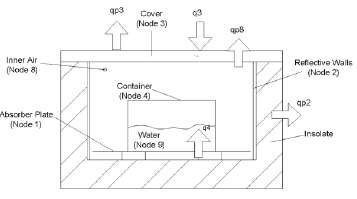Figure 2.
Schematic for illustrating the position of each node where the respective energy balance is made

The electric circuit analogy for the system that composes the container can be seen in Figure 2. In Figure 3, different node or energy exchanger surfaces, which are interconnected one to another by thermal resistance, can be seen.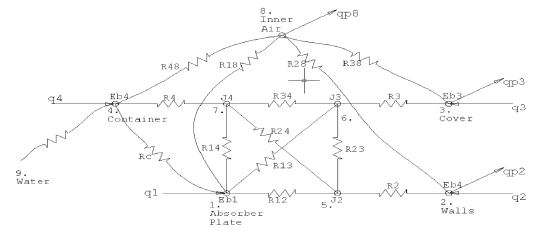Figure 3.
Electric circuit analogy built for box solar stove.

Each resistance corresponds to a different type of heat exchanger, e.g., radiation, convection, or conduction between the different surfaces. The expressions obtained for the energy balance according to the thermal system are:

Energy balance at absorber plate (node 1):

Each resistance corresponds to a different type of heat exchanger, e.g., radiation, convection, or conduction between the different surfaces. The expressions obtained for the energy balance according to the thermal system are:

• Energy balance at absorber plate (node 1):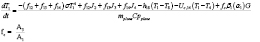(1)

• Energy balance at walls (node 2):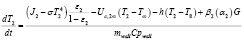(2)

• Energy balance at cover (node 3):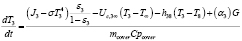(3)

• Energy balance at container (node 4):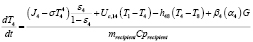(4)

• Energy balance at node 5: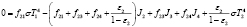(5)

• Energy balance at node 6: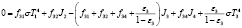(6)

• Energy balance at node 7: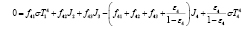(7)

• Energy balance at inner air (node 8):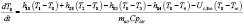(8)

• Energy balance at water (node 9):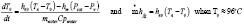(9)

The heat loss through the vertical walls of the stove is given by the following expression: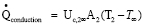(10)

Taking into account that the thermal resistance of the air surrounding the stove is small compared to the isolating layer resistance, the first is neglected and therefore the reflective walls just lose heat by conduction through the isolating material. The mechanisms of heat transfer by which the energy is lost through the cover are convection and radiation: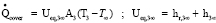(11)

The amount of energy that reaches each surface is influenced by properties such as transmissivity, reflectivity, as well as the direction of the incident radiation. Therefore, in these models i factor has been introduced to take into account these facts, whose value depends on the operating conditions.

To estimate the heat transfer coefficient due to convection in the enclosure, a maximum difference in temperature between the plate and the cover of 60°C is considered. Accordingly: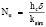Where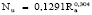and the Rayleigh number is given by: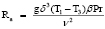, so Nusselt is established by Nu = 30.4 and the estimated transfer coefficient is h = 3.3 W/m2K. This would be the maximum value of the heat transfer coefficient due to convection in an enclosure that is being heated in the bottom part.

Two cases are treated to analyze the energy loss through the stove cover: Simple cover and double cover. Therefore, Ua1  2.6W/m2K and Ua2  1.75W/m2K are the heat transfer coefficients for simple and double cover, respectively.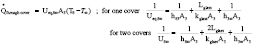(12)

To establish the heat transfer coefficient due to conduction between the receiver plate and the container, the following model is considered. An isothermal receiver plate is assumed during the process.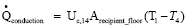(13)

SOLUTION METHOD

The model of each of the elements that physically constitutes the stove system through the energy balances has been stated to establish a set of equations that allows for the study of the temperature evolution in time, i.e., a set of ordinary differential equations. In addition, constitutive equations have been generated regarding optical and spatial surfaces properties and their configurations that have an algebraic form. This characteristic of mixing differential and algebraic equations is known as differential-algebraic equations (DAE), as follows:

x' = f(x, z, t) (14)

g(x,z,t)=0 (15)

Where x is the differential variable and z is the algebraic variable. The stated group of equations is known as a semi-explicit DAE system. The fact that this system is soluble by common methods depends on the system index that is related to the Jacobian singularity (if it exists). Because the Jacobian of these equations with respect to the algebraic variables (radiosities J) is non-singular, it is common to call this kind an index one system. Basically, there are two methods for solving these types of problems:

• Using the algebraic equations set to solve the algebraic variables (radiosities) in terms of the other variables, then putting in the differential equations to finally obtain an Ordinary Differential Equation (ODE) system.
• Using numerical methods developed to solve systems with these characteristics.

Matlab software contains pre-designed functions for index one DAE’s, this is the main reason to use it to simulate the solar stove heating .

5. EXPERIMENT

The solar radiation intensity was measured by using a pyranometer and a pyrheliometer during the test. On the other hand, to measure the temperature of different stove elements, thermocouples Type K for surface and immersion, a temperature tester for thermocouples, a thermometer, and a data acquisition system were also used.

Due to the operation conditions that affect the solar stove, the wind speed was one of the important variables registered using an anemometer. This process was carried out in order to evaluate the environment energy losses and it can be noted that the stove reached a temperature of over 60C in less than 30 minutes. It can be seen that the water boiling temperature is reached by the box stove (1 hour and 20 minutes).

6. RESULTS AND DISCUSSION

6.1 Instantaneous efficiency
In this case, the system efficiency is defined as the ratio of the energy gained by the water mass to the total energy supplied to the system. This is expressed as follows: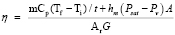(16)

The instantaneous efficiency is defined with the main purpose of observing how the variation occurs during the heating process.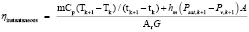(17)

The instantaneous efficiencies time evolution for different stove volumes is presented in Figure 4. In this figure, we can see how instantaneous efficiencies increase until they reach a maximum value, and then go down during the heating process with constant solar radiation (700 W/m2) and with the parameter shown in Table 1.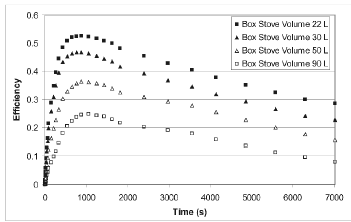Figure 4.
Instantaneous efficiencies for identical operating conditions: 700W/m2, 1Kg. water

Table 1. Value of the parameter used for the simulation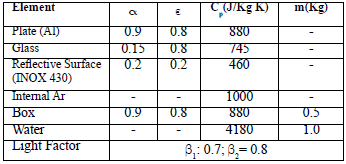We also can see that the instantaneous efficiencies are higher as the stove volume becomes higher too. These could be less if the stove were smaller, so it is possible to take more advantage of solar energy in a smaller cooker. In general terms, greater efficiencies do not mean (in this type of system) higher temperatures, but they imply the maximum extractable energy in a particular system.

6.2 Entropy Generation
Entropy generation will be evaluated in the stove system. To do this, an entropy balance at steady state is made: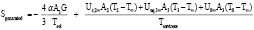(18)

To know how this entropy generation varies through the process, data obtained in the solution of systems in the transient state are used, and then this equation is solved at each time step by considering every one of these as quasi-steady states.

From Figures 5, it can be concluded that there is an inverse relationship between entropy generation and instant efficiency.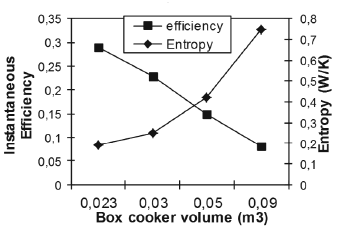Figure 5.
Instantaneous efficiency and entropy generation, evaluates different values in volume in a box stov

It was observed that for a box stove, as the box volume increases, efficiency decreases and there is more entropy generation.

6.3 Model validation
In order to validate theoretical results, a comparison was done between experimental and simulation temperature data, over the same operation conditions that were kept on this test, (See Figures 6a and 6b).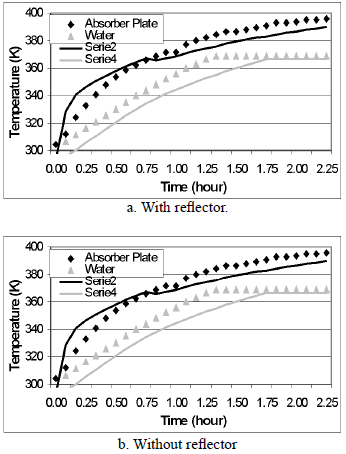Figure 6.
Experimental and theoretical temperatures evolution for box stove

We can say that theoretical and experimental temperatures behaviour is similar in both experiment with and without a reflector, and then a mathematical model is recommended for the use of anyone who wishes to make a design for this type of stove.

7. CONCLUSIONS

The system was designed to prepare food for human consumption using solar energy as the power source. Using the theoretical model and the simulation of performance, it was possible to study several solar stove configurations with different materials apt for application, and their thermal behaviour for many operation conditions. Making a comparison between the theoretical and experimental results, it was found that the simulation provides a closer and right prediction of the real thermal behaviour, for that reason, the mathematical-structured modelling is a reliable and precise tool for designing a solar stove.

ACKNOWLEDGEMENTS

The authors are grateful to the Universidad Nacional de Colombia through DIME for sponsoring, financial, and helping with the logistics for the successful development of this project.

NOMENCLATURE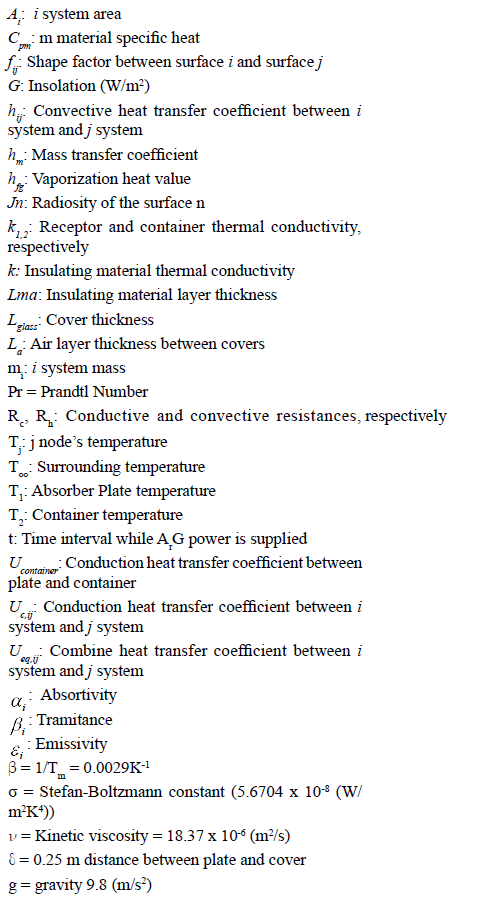REFERENCES

 ROSS, MC CLUNEY,. "Solar Oven Development and Testing". Florida Solar Energy Center. 2002.         [ Links ]
 FEUERMANN, D., GORDON, J.,. "Optical Performance of Axisymmetric Edge-ray Concentrators and Illuminators" Applied Optics, 37, (10), pp. 1905-1912. 1998.         [ Links ]
 RODRÍGUEZ, M. H., GONZÁLES B, F., "Manual de Radiación en Colombia", Bogotá, pp. 27. 1992.         [ Links ]
 ESTRADA, D.; MACIA., A. Diseño Construcción y Puesta en Operación de una Cocina Solar para la Preparación de Productos Alimenticios, TDG, Facultad de Minas, Universidad Nacional de Colombia. 2003.         [ Links ]
 KLEMENS S., VIEIRA DA SILVA M. E, "Characterisation and design methods of solar cookers". Solar Energy, Vol 82, Issue 2, pp. 157-163. February 2008.         [ Links ]
 PASSAMAI, V; TILCA, F. "Hot Box Solar Stove". Salta: La Universidad. Facultad de ciencia exactas, 2000.         [ Links ]
 MOHAMAD, M., ABDEL, K, A., "Analytical and experimental performance of hot box solar stove". Cairo: La Universidad: Facultad de ingeniería. 1998.         [ Links ]
 KLEMENS SCHWARZER, MARIA EUGÊNIA VIEIRA DA SILVA. "Characterisation and design methods of solar cookers". Solar Energy vol. 82, 157-163. 2008.         [ Links ]
 A.V. NARASIMHA RAO AND S. Subramanyam. 2005. Solar cookers-part-II-cooking vessel with central annular cavity. Solar Energy, vol 75, 19-22.         [ Links ]
 P. KARIUKI NYAHORO, RICHARD R. JOHNSON AND JOHN EDWARDS. "Simulated performance of thermal storage in a solar cooker". Solar Energy, vol. 59, 11-17. 1997.         [ Links ]
 JOHNSON, R., EDWARDS, J, KARIUKI P. "Simulated performance of thermal storage cooker". Solar Energy. Vol 59. p. 11-17. 1997.         [ Links ]
 WINSTON, R., 1994, "Optical Nonaimaging". Science and Investigation. Vol. 18. No. 62. p. 52-58.         [ Links ]
 MILLS, ANTHONY, F. Transferencia de calor. Santa Fé de Bogotá: Mc Graw hill, 1995.         [ Links ]
 JENKIN, D., WISTON, R., 1996. Integral desing method for nonimaging Concentrators. Optical Society of America. Vol.13, No.10; 2106-2112.All the contents of this journal, except where otherwise noted, is licensed under a Creative Commons Attribution License